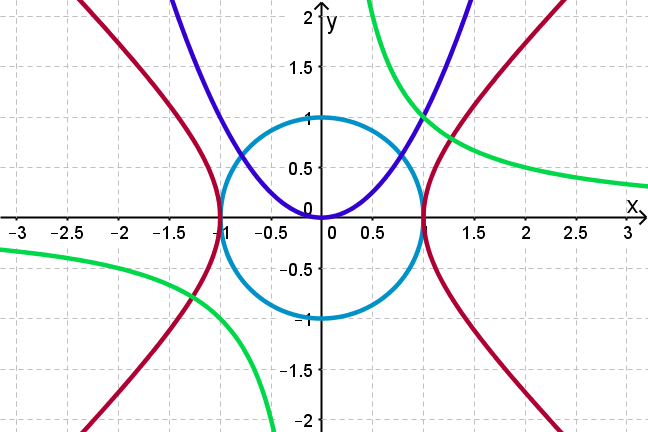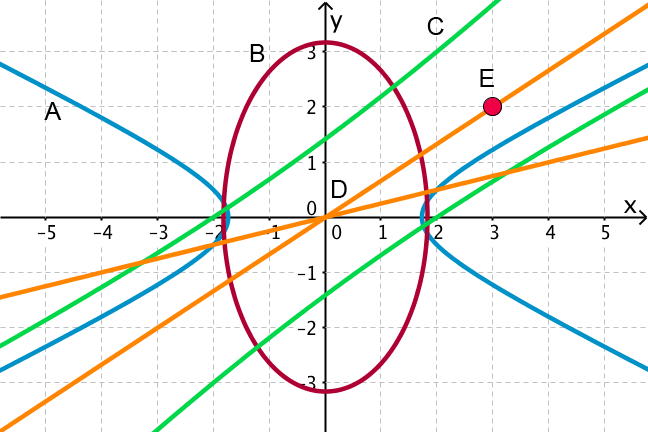## Want to keep learning?

This content is taken from the UNSW Sydney's online course, Maths for Humans: Linear, Quadratic & Inverse Relations. Join the course to learn more.
2.5

## UNSW SydneySome basic conics

# Conic sections as second degree curves

The crucial discovery that Descartes made about conics is that they exactly correspond to second degree equations, involving at least one of the terms $$\normalsize{x^2}$$, $$\normalsize{xy}$$ or $$\normalsize{y^2}$$. This is then the second step on a hierarchy of curves which begins with lines as linear equations. Algebra brings order to the world of curves!

In this step you will

• see some basic examples of conics as second degree curves

• get some experience with some more complicated equations for conic sections.

If you are interested more in the history, see Norman’s YouTube lecture on analytic geometry.

## Second degree equations

Descartes realised that a second degree polynomial equation in $$\normalsize{x}$$ and $$\normalsize{y}$$ like

$\Large{3x^2-xy+y^2-x+y=5}$

must be one of the conic sections studied by Apollonius. So if you randomly make up a polynomial equation involving the variables $$\normalsize{x}$$ and $$\normalsize{y}$$, with the maximum degree of any term being two, then this determines a curve which is a conic section of one type or another, somewhere in the plane.

Q1 (E): Which of these are second degree curves?

i) $$\normalsize{x^2-y^3=xy+1}$$

ii) $$\normalsize{5x^2-xy=6y^2+1}$$

iii) $$\normalsize{x=4y+7}$$

iv) $$\normalsize{x^2-3y=x+x^2+8}$$

v) $$\normalsize{x(x-y)-y(4y+3)=-1}$$.

## Some simple, common second degree curves

Let’s have a look at some of the most commonly occurring second degree equations and the curves they generate.The equation $$\normalsize{x^2+y^2 =1}$$ is the unit circle (light blue) with centre the origin $$\normalsize{O=[0,0]}$$. It has points $$\normalsize{[\pm 1,0]}$$ and $$\normalsize{[0,\pm1]}$$ lying on it, but also others obtained from Pythagorean triples, such as $$\normalsize{[3/5,4/5]}$$ and $$\normalsize{[5/13,-12/13]}$$.

The equation $$\normalsize{y=x^2}$$ is the standard parabola (dark blue), with points like $$\normalsize{[1,1]}$$ and $$\normalsize{[2,4]}$$ lying on it.

The equation $$\normalsize{xy=1}$$, or equivalently $$\normalsize{y=1/x}$$, defines a hyperbola (green) with asymptotes along the coordinate axes, meaning that the hyperbola approaches more and more closely these lines as you proceed along it. Of course the hyperbola has many points that can easily be generated on it, such as $$\normalsize{[3,1/3]}$$ and $$\normalsize{[1/4,4]}$$.

The equation $$\normalsize{x^2-y^2=1}$$ defines a hyperbola (red) with asymptotes lines of slope $$\normalsize{1}$$ and $$\normalsize{-1}$$.

Q2 (M): Find some points $$\normalsize{[x,y]}$$ on the hyperbola $$\normalsize{x^2-y^2=1}$$ for which neither $$\normalsize{x}$$ nor $$\normalsize{y}$$ are zero.

## Some more general examples

How about a more complicated equation, such as

$\Large{3x^2-2xy+y^2+2x-y-5=0}?$

This is still a second degree, or degree-two, curve. How could we possibly graph the points $$\normalsize{[x,y]}$$ that satisfy this equation? This is not an easy task, but a geometry/graphics program like GeoGebra has no problem: we just input the equation into the input line and see what nice picture emerges. This makes graphing complicated equations a lot simpler than it was in Descartes’ day!In this case we get an ellipse, also not quite centred in the usual spot, and tilted at some different angle. Can we see any points that lie on the ellipse? The integer point $$\normalsize{[1,3]}$$ looks like it might work, and if we plug it into the equation, we see that we really do get zero, so this is a bona fide point on the curve.

$\Large{ x^2+4xy-y^2+3x=6}?$

GeoGebra shows us this is a hyperbola with centre near $$\normalsize{[0,0]}$$, and tilted at some angle.In general, deciding what kind of a curve a general quadratic equation gives is beyond the scope of this course. What we want you to know is that it is always going to be a conic section.

## Pairs of lines

There is a degenerate case that we need to mention. We saw in a previous step that something special happens if the plane cutting the cone passes through the apex of the cone. In that case the plane could cut out a pair of lines, or perhaps just a single line, or perhaps just a single point.

Can you visualise the graphs that would form from these different possibilities (making yourself a model of a cone might help!)?

Here is an example of a second degree equation with this degenerate kind of character:

$\Large{2x^2+xy-15y^2=0.}$GeoGebra tells us that this is a pair of lines. In fact this is also a conic section, and in the language of Apollonius would correspond to slicing a cone with a plane that passes through the vertex of the cone.

Q3 (M): By using a bit of algebra, explain why the graph above is pictorially a pair of lines. (In other words, we could have deduced this special case without any appeal to pictures!)

Now let’s observe what happens when we change the constant term on the right hand side of the equation. Below we see the graphs of

$\Large{(x+3y)(2x-5y)=1}\quad\text{ and }\quad{(x+3y)(2x-5y)=-1}$

which are the blue and red hyperbolas respectively, with asymptotes the original pairs of green lines. So the “pair-of-lines” conic might be thought of as a special, or degenerate case of the hyperbola.Q4 (M): By looking at special points, or comparing to standard equations, or just by hook or crook, see if you can match up these equations to the associated conics in the figure below.

i) $$\normalsize{x^2-4y^2=3}$$

ii) $$\normalsize{2x^2-11xy+12y^2=0}$$

iii) $$\normalsize{x^2+y^2-6x-4y+13=0}$$.

iv) $$\normalsize{3x^2+y^2=10}$$

v) $$\normalsize{x^2-3xy+2y^2=4}$$.A1. i) $$\normalsize{x^2-y^3=xy+1}$$ is not second degree since the term $$\normalsize{y^3}$$ is of degree three, ii) $$\normalsize{5x^2-xy=6y^2+1}$$ is a conic since there are terms of degree two but no higher, iii) $$\normalsize{x=4y+7}$$ is a linear equation, which does not count as a quadratic equation, iv) $$\normalsize{x^2-3y=x+x^2+8}$$ appears to be second degree but in fact the $$\normalsize{x^2}$$ terms cancel, so it is actually of degree 1, and v) $$\normalsize{x(x-y)-y(4y+3)=-1}$$ is a second degree curve. Second degree curves are also called quadratic.

A2. Here are some points: $$\normalsize{[5/4,3/4]}$$, $$\normalsize{[13/5,12/5]}$$, $$\normalsize{[17/8,15/8]}$$. Perhaps you can guess some others?

A3. Algebraically we can see that the equation $$\normalsize{2x^2+xy-15y^2=0}$$ is a pair of lines by factoring it:

$\Large{2x^2+xy-15y^2=(x+3y)(2x-5y)=0.}$

If we recall that a product of two terms equals $$\normalsize{0}$$ precisely when either or both of the terms is zero, we see that this equation is really equivalent to the pair of equations $$\normalsize{x+3y=0}$$ or $$\normalsize{2x-5y=0}$$ , and since each of these linear equations determines a line, the graph is indeed a pair of lines.

A4.

i) Let’s consider this equation when $$\normalsize{x=3}$$. Then $$\normalsize{y^2 = 3/2}$$, and so $$\normalsize{y=\pm\sqrt{3/2}\approx \pm 1.22}$$ approximately. The only curve which passes close to the approximate points $$\normalsize{[3, \sqrt{3/2}]}$$ and $$\normalsize{[3, -\sqrt{3/2}]}$$ is the blue hyperbola A.

ii) The origin $$\normalsize [0,0]$$ satisfies the equation for this curve, so this must be the orange pair of lines D.

iii) This is an equation for the point E, namely $$\normalsize{[3,2]}$$. Note that the equation reduces to $$\normalsize{(x-3)^2 + (y-2)^2} = 0$$, which is the algebraic form of a circle with radius $$\normalsize 0$$. So points, like pairs of lines, can be viewed as degree-two equations!

iv) The equation $$\normalsize{3x^2+y^2=10}$$ is the red ellipse B, since values of $$\normalsize x$$ and $$\normalsize y$$ bigger than $$\normalsize 4$$ are impossible.

v) Let’s consider the equation when $$\normalsize{x=0}$$. Then $$\normalsize{y^2 = 2}$$, and so $$\normalsize{y=\pm\sqrt{2}\approx \pm 1.41}$$ approximately. The only curve which passes close to the approximate points $$\normalsize{[0, \sqrt{2}]}$$ and $$\normalsize{[0, -\sqrt{2}]}$$ is the green hyperbola C. Even though this might appear to be a pair of lines, it is actually a hyperbola.